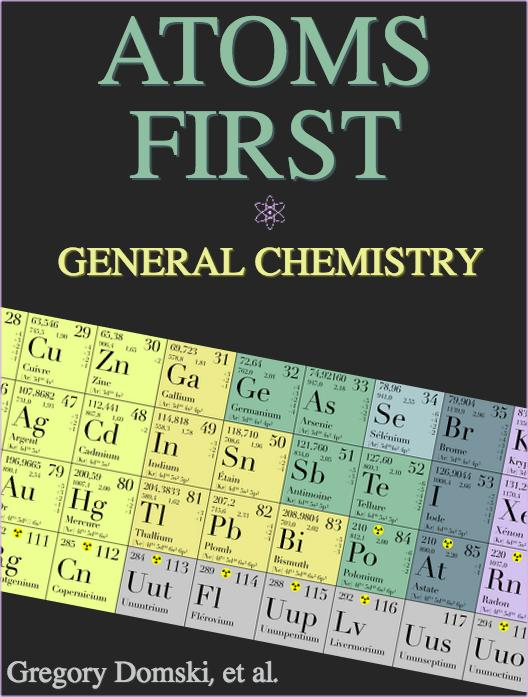# Atoms First General Chemistry

This book helps students understand atoms, ions, and molecules conceptually before they encounter the topics of stoichiometry, kinetics, and thermodynamics.

## What is a Top Hat Textbook?

Top Hat has reimagined the textbook – one that is designed to improve student readership through interactivity, is updated by a community of collaborating professors with the newest information, and accessed online from anywhere, at anytime.

• Top Hat Textbooks are built full of embedded videos, interactive timelines, charts, graphs, and video lessons from the authors themselves
• High-quality and affordable, at a significant fraction in cost vs traditional publisher textbooks

## Key features in this textbook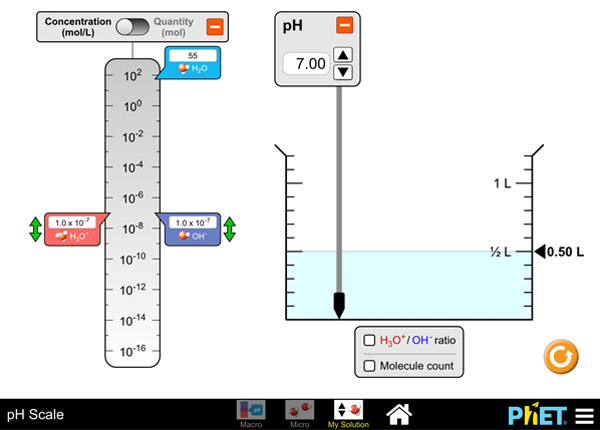Atoms First Chemistry engages students with all different learning styles, offering plentiful visuals, instructional videos and interactive simulations.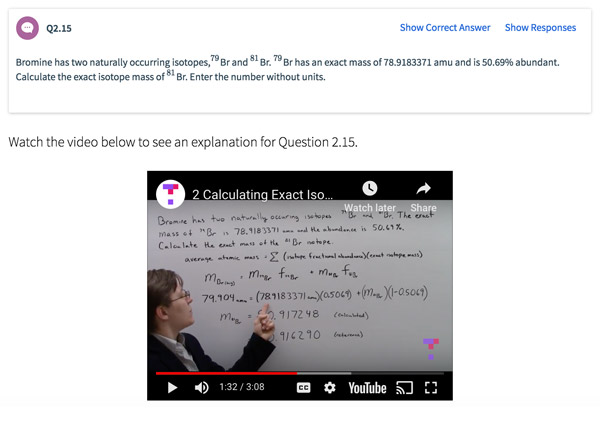Built-in assessment questions embedded throughout chapters so students can read a little, do a little, and test themselves to see what they know!

## Comparison of General Chemistry Textbooks

Consider adding Top Hat’s Atoms First General Chemistry to your upcoming course. We’ve put together a textbook comparison to make it easy for you in your upcoming evaluation.

### Top Hat

Greg Domski, et.al, “Atoms First General Chemistry”, Only One Edition needed

### McGraw-Hill

Julia Burdge, et.al, Chemistry: Atoms First, 2nd Edition

### Nelson Education

Zumdahl, et al, Chemistry: An Atoms First Approach

### Pricing

Average price of textbook across most common format

E-book

### Always up-to-date content, constantly revised by community of professors

Content meets standard for American Government. Constantly revised and updated by a community of professors with the latest content

### In-Book Interactivity

Includes embedded multi-media files and integrated software to enhance visual presentation of concepts directly in textbook

Only available with supplementary resources at additional cost

### Customizable

Ability to revise, adjust and adapt content to meet needs of course and instructor

### Built-in interactive assessment questions

Assessment questions with feedback embedded throughout textbook

## Pricing

Average price of textbook across most common format

### Top Hat

Greg Domski, et.al, “Atoms First General Chemistry”, Only One Edition needed

### McGraw-Hill

Julia Burdge, et.al, Chemistry: Atoms First, 2nd Edition

E-book

## Always up-to-date content, constantly revised by community of professors

Constantly revised and updated by a community of professors with the latest content

### Top Hat

Greg Domski, et.al, “Atoms First General Chemistry”, Only One Edition needed

### McGraw-Hill

Julia Burdge, et.al, Chemistry: Atoms First, 2nd Edition

### Nelson Education

Zumdahl, et al, Chemistry: An Atoms First Approach

## In-book Interactivity

Includes embedded multi-media files and integrated software to enhance visual presentation of concepts directly in textbook

### Top Hat

Greg Domski, et.al, “Atoms First General Chemistry”, Only One Edition needed

### McGraw-Hill

Julia Burdge, et.al, Chemistry: Atoms First, 2nd Edition

### Nelson Education

Zumdahl, et al, Chemistry: An Atoms First Approach

## Customizable

Ability to revise, adjust and adapt content to meet needs of course and instructor

### Top Hat

Greg Domski, et.al, “Atoms First General Chemistry”, Only One Edition needed

### McGraw-Hill

Julia Burdge, et.al, Chemistry: Atoms First, 2nd Edition

### Nelson Education

Zumdahl, et al, Chemistry: An Atoms First Approach

## All-in-one Platform

### Top Hat

Greg Domski, et.al, “Atoms First General Chemistry”, Only One Edition needed

### McGraw-Hill

Julia Burdge, et.al, Chemistry: Atoms First, 2nd Edition

### Nelson Education

Zumdahl, et al, Chemistry: An Atoms First Approach

#### Greg Domski, Ph.DAugustana College, Illinois

Greg Domski received his M.S (2005) and Ph.D (2008) in Inorganic chemistry from Cornell University, Ithaca, NY. He is currently an Associate Professor of Chemistry at Augustana College in Illinois, teaching General, Organic and Inorganic chemistry. His research interest include the development of novel binuclear donor-functionalized N-heterocyclic carbene transition metal complexes for transfer hydrogenation catalysis and the development of transition metal-based olefin polymerization catalysts. Greg also works closely with his students in developing their research and presenting their findings.

## Explore this textbook

Read the fully unlocked textbook below, and if you’re interested in learning more, get in touch to see how you can use this textbook in your course today.

# Chapter 1: Matter and EnergyChemistry has applications in many different fields. Examples can be found in the disciplines of medicine, food, materials engineering, environmental science, and many more. Chemistry and chemical processes can be found anywhere on the planet. After reading this text, we think you'll agree that chemical processes play a major role in driving the natural world. 

## Learning Objectives

By the end of this chapter, you should be able to:

• Differentiate between the three states of matter.
• Explain how matter can be identified by its composition on the atomic level.
• Identify physical and chemical changes that matter can undergo.
• Identify all stages of the scientific method.
• Know the (Système International) SI units for common physical quantities.
• Understand and use prefix multipliers.
• Identify and use significant figures in calculations; understand how they convey precision.
• Use dimensional analysis to convert between units.
• Use Avogadro's number to convert between the number of atoms/ions/molecules in a sample and the number of moles.
• Estimate the relative strength of Coulombic potential energy between charged particles.
• Classify common phenomena according to the type of energy driving them.

## 1.1 What is Chemistry?

In the opening scene of the first episode of Breaking Bad, the primary character, Walter White, defines chemistry as “the study of matter…the study of change.” He sprays solutions into the flame of a Bunsen burner during his high school chemistry class. The yellow flame immediately turns different colors. He then proclaims that “electrons change their energy levels, molecules change their bonds, elements combine and change to compounds…that’s all of life…it is truly fascinating…” In this scene, Walter captured the essential focus of all branches of natural and physical science: matter, energy, and the changes they undergo.

Each branch of the natural and physical sciences (e.g., biology, physics, geology, chemistry) deals with matter, energy, and change. For example, biologists focus on the study of living matter (organisms) and how it uses energy (e.g., food, sunlight, etc.) to change (i.e., grow). Geologists study terrestrial matter (e.g., rocks and soil) and how energy affects it (e.g., how gravitational energy creates pressure that results in to formation of different minerals).

While biologists focus on living matter and geologists study terrestrial matter, chemists study the fundamental building blocks of matter: atoms, ions, and molecules.

Chemistry is the study of matter, its properties, and the mechanisms involved in its changes. In this introductory chapter, we’ll first explore what we mean by matter and how to characterize the three different states. Then, we’ll address the process scientists use to investigate matter, its properties, and changes. Next, we'll develop the skills needed to work with measured quantities which serve as the basis of the modern scientific method. Finally, we will discuss the various forms of energy and focus on the types of energy that are most relevant to the study of chemistry.

## 1.2 The Properties and Transformations of Matter

### 1.2.1 Matter and Its States

Chemistry is the study of changes in energy and the properties of matter.

Matter is any substance that has mass and occupies space. The fundamental building blocks of matter are atoms, which cannot be chemically broken down into smaller units. The word “atom” has its origins from the Greek word “atomos,” which means “uncuttable.” Think of atoms as individual Lego pieces. Atoms can combine to form larger molecules and compounds. The three traditional forms or states of matter are solids, liquids, and gases.

Solids are characterized by having a defined shape, a defined volume, and are not compressible (that is, they are difficult to press into a smaller volume). Atoms within solids tend to be relatively immobile and in constant contact with adjacent atoms. These characteristics help to give solids their mostly rigid nature.

Liquids are characterized by having a definite volume, no definishape, and are not compressible. Atoms within liquids tend to have a greater degree of motion than in solids. This helps to explain why liquids have no shape and are freely flowing.

Gases are characterized by having neither definite shape nor definite volume. In fact, gases will assume the shape and volume of whatever container they occupy. Gases are easily compressible, which is a major difference from solids and liquids. In the gaseous state, atoms are at relatively far distances from each other and travel in random directions at very high velocities. This helps explain why gases are difficult to contain and quickly fill an entire space. The properties of the states of matter are summarized in Table 1.1.

​Since atoms are the fundamental building block of matter that chemists are interested in, it makes sense to consider their basic structure now. In Chapters 2 and 3, we will take a much more detailed view of atomic structure. For now, it is important to know the following:

1. Atoms are incredibly small. For example, a sheet of aluminum foil is about 200,000 atoms thick.

2. Atoms are made of three subatomic particles: neutrons, protons, and electrons (see Figure 1.2). The center of the atom is called the nucleus; the neutrons and protons reside here. The nucleus is positively charged due to the fact that protons bear a positive charge. Most of the space an atom takes up is made up of fast-moving, negatively-charged electrons that are attracted to the nucleus. Atoms are typically depicted as spheres (Figure 1.1).​Figure 1.2. Nuclear model of the atom in the early 20th century showing locations of all subatomic particles.​ Note: particles are not drawn to scale.

3. The number of protons in an atom's nucleus determines the identity of the element. For example, gold (Au) has 79 protons in its nucleus while silver (Ag) has 47 (Figure 1.3)

Q1.01

Pair each trait with the appropriate physical state.

Premise
Response
1

Solids

A

Have volume but no shape

2

Liquids

B

Are highly dispersed

3

Gases

C

Atoms closely packed together

Commonly used illustrations for atoms and molecules are called “particulate-level drawings.” Small spheres represent individual atoms while different colors represent atoms of different elements. The following figures depict the three states of matter. Note the relative spacing given in each diagram. In addition, gas atoms usually have a gradient color scheme used to indicate their higher speeds.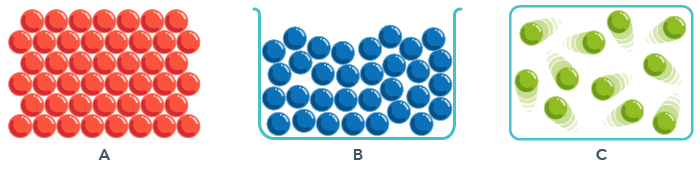Figure 1.4. A) Arrangement of atoms in a solid B) Arrangement of atoms in a liquid C) Arrangement of atoms in a gas.

### 1.2.2 The Composition of Matter

Matter can be divided into two categories: pure substances and mixtures. A pure substance is made up entirely of one specific arrangement of atoms/molecules and cannot be physically separated. Pure substances can be subdivided further into elements and compounds. An element is made up of only one type of atom. Elements cannot be chemically broken down into smaller atoms. An example is sodium metal, shown in Figure 1.6.

Sodium is an element because the smallest repeatable unit is an individual sodium atom. A compound combines more than one element into its smallest repeatable unit. Compounds can be broken down into smaller, individual elements. Examples are glucose (C6H12O6), carbon dioxide (CO2), and propane (C3H8).

Q1.02

Identify the following as either an element or a compound.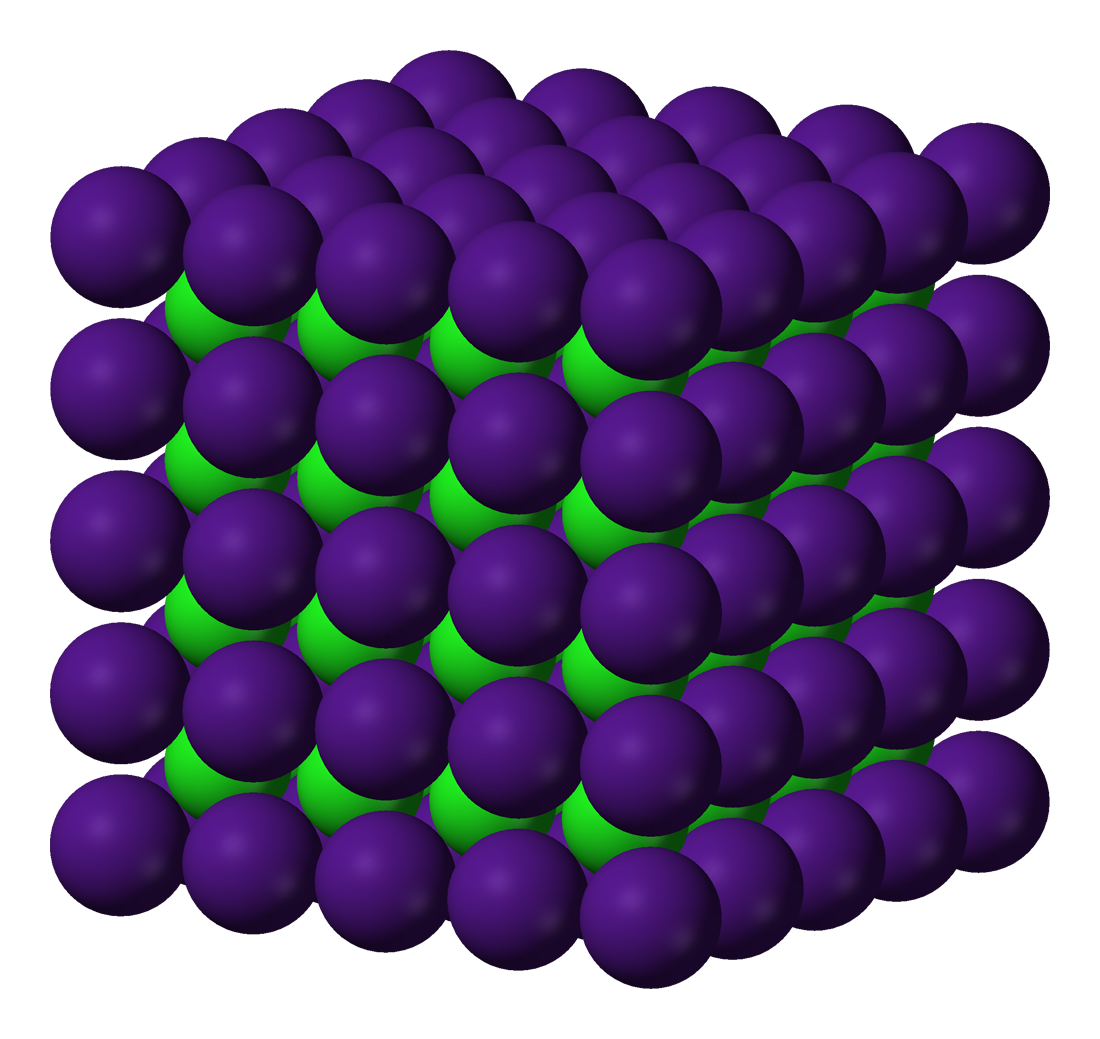A

Element

B

Compound

Q1.03

Identify the following as either an element or a compound.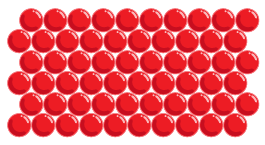A

Element

B

Compound

Mixtures contain more than one compound. An example is ocean water, which contains primarily two compounds: salt (NaCl) and water (H2O). Mixtures are either homogeneous or heterogeneous as shown in Figure 1.7.

Homogeneous mixtures have a uniform consistency, which means that all the compounds in the mixture are regularly distributed. The majority of beverages are homogeneous mixtures, such as juices and sports beverages. Every sip of these beverages has the same taste and texture. Heterogeneous mixtures have a non-uniform consistency, which means the compounds in the mixture are not equally dispersed throughout the sample. A typical example is a mixture of oil and water.Figure 1.8. A heterogeneous mixture of oil on top of water. 
Q1.04

Identify the following as either a homogeneous or heterogeneous mixture.A

Homogeneous mixture

B

Heterogeneous mixture

Q1.05

Identify the following as either a homogeneous or heterogeneous mixture.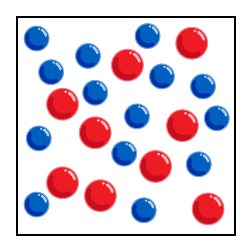A

Homogeneous mixture

B

Heterogeneous mixture

Q1.06

Identify each figure as an element, compound, homogeneous mixture, or heterogeneous mixture.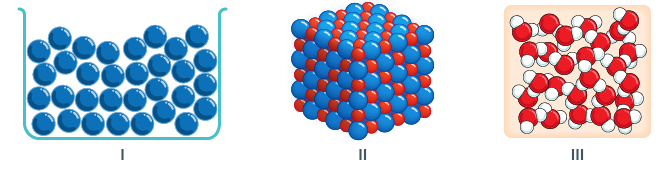Premise
Response
1

I

A

Compound

2

II

B

Homogeneous mixture

3

III

C

Heterogeneous mixture

D

Element

E

Compound

### 1.2.3 The Properties and Changes of Matter

As we said at the beginning of the chapter, chemistry is the study of matter, its properties, and the changes that it undergoes. Now that we have a fundamental understanding of the forms and composition of matter, let’s address the physical and chemical properties of matter. Physical properties are traits or characteristics that can be expressed without requiring a change in chemical composition or identity. Physical properties can often be described by our senses. These include odor, texture, color, and shape. In addition, physical properties can often be described with a measurement. Examples include mass, volume, melting point, boiling point, and density (discussed at the end of this chapter). Chemical properties are traits or characteristics that can be expressed only after a change in chemical composition has occurred. Examples include the tendency to react with oxygen (i.e., oxidative ability), flammability, acidity, basicity, corrosiveness, and spoiling.

Q1.07

Identify each of the following as a physical or chemical property.

Premise
Response
1

Sand is coarse

A

Chemical

2

Fruit easily turns brown when exposed to air.

B

Physical

C

Physical

D

Chemical

Physical changes are transformations that do not involve a change in chemical composition and can easily be undone (reversible). Examples include any phase changes, such as melting, evaporation, freezing, and condensation. Another common physical change involves the simple mixing of a solid with a liquid (i.e., dissolving): when salt is combined with water, the chemical identities of both the salt and water are retained.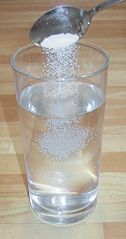Figure 1.10. Dissolving salt in water is a physical change. 

​ Chemical changes involve a change in chemical composition. Atoms and molecules get redistributed and end up in a different combination than when they started. For example, the reaction between iron (Fe) and oxygen (O2) results in the formation of rust (Fe2O3). Chemical changes are often irreversible; that is, they cannot be undone. Everyday examples include frying an egg, burning toast, and baking a cake. Rusting is a chemical process involving the oxidation of iron metal ​.Figure 1.11. Rusting is a chemical process involving the oxidation of iron metal. ​​​

Q1.08

Identify each of the following as a physical or chemical change.

Premise
Response
1

Sugar mixes very well with coffee.

A

Physical

2

Gaseous vapor observed when handling dry ice (solid CO$_2$).

B

Chemical

C

Chemical

D

Physical

## 1.3 The Scientific Method

Everyone solves problems on a daily basis. Why isn’t my Wi-Fi working? Why isn’t my car starting? Why am I getting an upset stomach? The general procedure we use to solve problems might be:

• Identify or observe the problem.
• Try to make an educated guess as to the cause.
• Try to run a few tests to see if your guess is right or not. If after the tests you decide your initial guess was incorrect, and then try to come up with another possible explanation and test it too.
• Try to come up with a general statement that can be used to describe the original problem: causes, reasons, and expected situations when the problem could arise again.

Consider a 6-month old baby who starts a diet of solid foods. The baby eats some meat, some vegetables, and some fruit. After a few days, the baby develops a rash. The parents obviously want to solve this problem as quickly as possible. The pediatrician suggests they feed the baby only one type of food at a time and monitor the baby’s skin for a week. If all is clear, introduce a new food item and repeat. By changing only one item at a time, the cause of the allergy can be pinpointed.

Q1.09

Is it absolutely certain that food caused the allergy?

A

Yes

B

No

That’s right. The probable cause is food, but there could be many other factors, such as a new type of clothing, soap, shampoo, etc. We’ll return to this later when revision of the original guess is necessary.

Moreover, it isn’t 100% certain that one food item is the cause. It could be a combination of foods, or simply a stage of life the baby is experiencing. In other words, the main finding is not necessarily the bottom line.

The scientific method is the process scientists use to identify, investigate, and solve problems. The first step is to record one’s own observations. Qualitative observations are descriptive and do not include measurements or values. Examples include color, texture, odor, etc. Quantitative observations come from measurements, including weight, length, temperature, etc. In the example about the baby, the parents qualitatively observed an allergic reaction.

Afterward, the parents/pediatrician suggested that the reaction was due to the introduction of solid food. They formed a hypothesis, which is an educated guess that provides a cause of the main observation.

The next stage was to carry out tests via experimentation. The parents only introduced one type of food at a time. In other words, they changed one variable at a time and monitored the change, if any. This latter part involves data collection.

The results were then analyzed to see if they showed any trends. Did the results suggest that food was the culprit? This is where the earlier hypothesis would either be confirmed or refuted. If the hypothesis turned out not to be true, then a new statement should be formulated followed by further experimentation and data analysis.

Since the removal of meat coincided with the baby being allergy-free, the parents concluded that that baby was allergic to meat. In science, a theory is a tested, unifying statement that explains a series of observations. Keep in mind that a theory is only true until proven otherwise. For example, the baby could grow out of his/her allergy to meat.

The words “law” and “theory” often get confused. A law is a conceptual or mathematical statement about what is observed in nature that has been proven true. Laws are generally considered to be fact but do not offer an explanation for the underlying reasons. A theory is an explanation of a scientific law based on the results of many experimental observations and can link several laws together to suggest an underlying theme or principle. Like hypotheses, theories are subject to revision if compelling new evidence is discovered.

Q1.10

Identify each statement as either a law or a theory.

Premise
Response
1

Two bodies attract each other with a force that is directly proportional to their masses but inversely related to their distance.

A

Theory

2

Through a process known as “panspermia” micro-organisms on asteroids crash landed on Earth 4 billion years ago thus seeding the planet with the origins of life

B

Law

## 1.4 The Units of Measurement

In science, most quantities that are calculated or measured in the laboratory have a unit following them. A number is meaningless unless a unit is provided to indicate what property you are describing! Unfortunately, two systems are currently in use, which can be inconvenient. In the United States, the English system is used, while the rest of the world uses the metric system. Many units comprise the metric system, but one standard subset has been adopted in the scientific community called SI units (Système International).

For mass, the English unit is the pound (lb.), while the SI unit is the kilogram (kg). Mass is the amount of matter in a substance. From a physics standpoint, it is a measure of a substance’s resistance to acceleration. A common misconception is the relationship between mass and weight. The latter represents a substance’s attraction to a nearby body. On Earth, a person’s weight represents his/her gravitational attraction to Earth.

For length, the English unit is the yard (yd) and the SI unit is the meter (m).

For volume, the English unit is the gallon (gal) and the SI unit is the cubic meter (m3). Volume is the amount of three-dimensional space a substance occupies.

For temperature, the English unit is degrees Fahrenheit (°F), whereas the rest of the world uses degrees Celsius (°C). You can see when traveling abroad that this dual system is an inconvenience! When this author traveled abroad for the first time, he wore warm clothes for a 37°C day (98.6°F)!

The SI unit for temperature is the Kelvin (K). There’s often confusion between “temperature” and “heat.” Temperature is a measurement of the kinetic energy of molecules inside a sample. The more motion that occurs, the higher the temperature. On the other hand, heat is a transfer of energy due to a temperature difference. Heat is often associated with the loss or gain of “thermal energy” between a system and its surroundings (more on this in Chapter 5).

The following equations allow for conversion between temperature units:

Kelvin is often referred to as an absolute scale since the lowest possible theoretical value is zero Kelvin (absolute zero). Also, notice that two of the three temperature units are identically scaled.

Q1.11

Kelvin and _______ are on identical scales.

A

°C

B

°F

To conveniently express a very small or very large measured value, a system of prefix multipliers exists, as shown in Table 1.2.

The “base unit” is the unit without any prefixes attached (e.g., L instead of mL, m instead of km, g instead of mg). All prefixes on top of the “base unit” row represent larger scales than the base. For example, 1 kilogram equals 103 = 1000 grams. All prefixes below the base unit row represent smaller scales than the base. For example, one milliliter equals 10-3 Liters.

Q1.12

Rank the following in order of decreasing length.

A

100 m

B

100 nm

C

100 km

D

100 cm

A common abbreviation that is used for volume in a medical setting is “cc,” which stands for cubic centimeter (1 cm3 = 1 cc = 1 mL).

## 1.5 Review of Scientific Notation

Scientific notation is used to conveniently express very large or very small numbers. A value expressed this way is written in the following format:

If the integer is negative, then the overall quantity is less than 1. If the integer is positive, then the overall quantity is greater than 1.

The integer tells us how many places to move the decimal point when converting from scientific notation to standard numerical format. If the integer is negative, then the decimal is moved to the left that many places. If the integer is positive, then the decimal is moved to the right.

Q1.13

Convert 1.145 × 10$^5$ to decimal format.

Q1.14

Convert 4.653 × 10$^{-6}$ to a decimal number.

When going the reverse direction, the decimal movement is opposite of what we just did! Remember that the goal is to move the decimal point to achieve a value ≥ 1, but less than 10. If you have to move the decimal point to the right, then the integer is negative.

Q1.15

Convert 0.0003215 to scientific notation. Use “E” for “×10” followed by the power.

If you have to move the decimal point to the left, then the integer is positive.

Q1.16

Convert 4356.143 to scientific notation. Use “E” for “×10” followed by the power.

## 1.6 Accuracy and Error

The boiling point of water is 100.0°C at sea level. Suppose you used a thermometer to measure a beaker of boiling water in the lab at sea level. The thermometer reads 99.8°C. Your measurement is close, but not exactly equal to the known boiling point of water. In science, we like to describe the accuracy of a measurement. Accuracy represents how close you got to a known, accepted value. A traditional analogue is how close you were able to “hit the bulls-eye.” Percent error is often included in laboratory reports and is a commentary on the accuracy of your measurement(s):

Q1.17

## 1.7 Significant Figures: A Measure of Precision

Consider the following two rulers:The bottom ruler has fewer increments than the top ruler. If we had 20 students make the same measurement indicated by the dashed line, which ruler would give the more reproducible result?

Q1.18

Which ruler would give more detailed results?

A

Top

B

Bottom

We’d likely get the same measurement repeated among the group of 20 students with the top ruler. We say that there is a higher level of precision using the ruler on the top. Precision describes the reproducibility of a measurement and is strictly determined by the quality of the instrument. Figure 1.15 illustrates the difference between accuracy and precision.

How could the ruler on the top be read? According to the ruler, the indicated distance is greater than 2.5 cm but less than 2.6 cm. This is certain and indisputable. So how should you record your measurement?

Q1.19

Is 2.5 cm correct?

A

Yes

B

No

Q1.20

A

Yes

B

No

Q1.21

A

Yes

B

No

It appears that there are multiple possible answers of the format “2.5___ cm.” The ruler on the top does not provide us information about the one-hundredths digit. Only the tenths digit is provided, and the hundredths digit is estimated by you. The rule of thumb is to report your measurements one digit past the last digit provided by the instrument. This is true when using non-electronic (analog) devices, such as glassware, rulers, and thermometers. We say that the final digit in 2.5____ cm is the digit of uncertainty, or the estimated digit.

Q1.22

Which digit is uncertain in the measurement: 23.147 meters?

A

2

B

3

C

1

D

4

E

7

Q1.23

What is an appropriate measurement, in centimeters, for the following pencil? Enter the numerical value without units.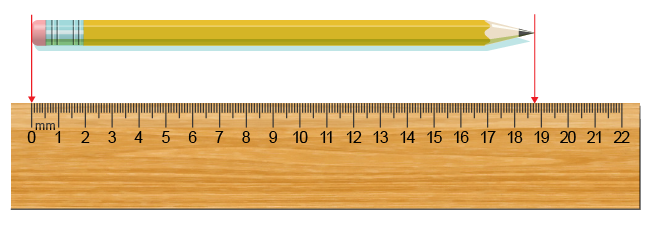Watch the video below for an explanation of the answer to Question 1.23.

Q1.24

What is an appropriate measurement for the following thermometer? Use the scale on the left side for °C. Go by the very top of the red level. Enter the numerical value without units.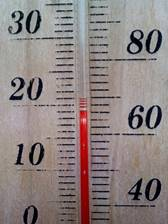Let’s give the next one a try.

Q1.25

What is an appropriate measurement for the following graduated cylinder? Enter the numerical value without units. You may need to zoom in using browser controls in order to read the volume.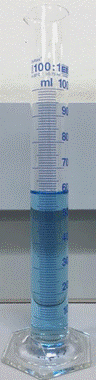What we can see from the preceding examples is a general trend.

Q1.26

The ______ the number of digits in a measurement, the more precise the reading.

A

Larger

B

Smaller

That’s right. The more, the better! These digits that affect a measurement’s precision are significant figures, or “sig figs” for short.

Here are the rules for identifying sig figs:

• All nonzero digits are significant, including all digits for a value expressed in scientific notation. For example, in the number 432 there are three sig figs. In 1.1451×105 there are five sig figs.
• A zero is significant if it is between nonzero digits. For example, in the number 50002, all five digits are significant.
• A zero is significant if it is a trailing zero to the right of the decimal point. For example, in the number 432.00, all five digits are significant.
• A zero is not significant if it comes at the end of a number without a decimal. For example, in the number 4130, the zero does not count and there are three sig figs, not four.
• Leading zeroes are not significant. For example, in 0.0004312, none of the zeroes are significant and there are only four sig figs.

Try the following problems.

Q1.27

How many sig figs are in 0.00034210?

Q1.28

How many sig figs are in 300.123940?

Let’s now discuss what is meant by infinite precision. This refers to what are called exact numbers. For example, when we say 20 students, we strictly mean 20 students, not 19.5 or 20.1. In other words, “20 students” is an exact quantity and has infinite precision. There is absolutely no uncertainty in this measurement! This applies to any countable quantity. Another case arises when an equality or equivalence statement is used. Consider 1 in. = 2.541 cm. This is a very precise quantity. Exactly 2.541 cm, not 2.54 cm or 2.542 cm, equals one inch.

Next, we’ll examine how sig figs are accounted for in calculations. For addition and subtraction, the rule is:

The number of decimal places in the final answer equals the fewest number of decimal places from the combined numbers.

For example, 1.34561 – 0.341 = 1.00461. However, there are only three decimal places in 0.341. Therefore, in 1.00461, the one-thousandths digit is the last digit that should be included in the final answer. So, do we keep the four or round up? If the number to the immediate right is <5, then we keep the four. If the number is ≥ 5, then we round up. Hence, 1.00461 rounds to 1.005.

Q1.29

Carry out the following calculation with the correct number of sig figs. 431.00791 + 52.5441

For multiplication and division, the rule is:

The number of sig figs in the final answer equals the fewest number of sig figs present from the contributing factors.

For example, 3.451 × 0.00671 = 0.02315621. The smaller number of sig figs from the contributing factors is three, from 0.00671.

Q1.30

Report 0.02315621 to three sig figs.

Finally, we’ll wrap up the calculations with problems concerning combined operations. The rule is:

Carry all digits until the end. “Tag” estimated digits after each step, but do not round until the very end.

Consider (1.32451 – 0.341)(1.0054). Here’s the raw answer after each step:

Let’s go back to (1.32451 – 0.341) and “tag” the last digit we would have kept. Because this is subtraction, we go by:

Q1.31

Let’s go back to (1.32451 – 0.341) and “tag” the last digit we would have kept. Because this is subtraction, we go by:

A

Fewest sig figs

B

Fewest decimal places

Q1.32

We keep all digits, but “tag” the third significant figure from the left: 0.98351

Next, 0.98351(1.0054) = 0.9888209554. The last operation is multiplication, so we should go by:

A

Fewest sig figs

B

Fewest decimal places

This is where the “tag” comes into play. We are stating that 0.98351 actually has three sig figs, not five! Hence, the raw answer 0.9888209554 rounds to 0.989.

Give it a go!

Q1.33

Carry out the following calculation with the correct number of sig figs. (431.00791 + 52.5441)/45.3200130

One more time:

Q1.34

Carry out the following calculation with the correct number of sig figs. (24.324)(3.2122) – 9.3210

## 1.8 Problem-Solving: Dimensional Analysis

Recently, this author visited Canada from the United States. At the time, gasoline cost $2.70/gallon in the U.S. and 100.0 cents/liter in Canada (assume a 1:1 exchange rate for simplicity). As you could imagine, he was very confused when it came time to pay for his fuel! You can see that knowing how to convert between different units can come in handy (we didn’t always have mobile devices to do this on the spot)! The method we use for unit conversion is called dimensional analysis, which follows the workflow: Unit A → Unit B → Unit C As you can see, it’s a matter of cancelling units until we have the “last unit standing.” The ratios that relate two different units are called conversion factors. For example, 1 gal = 3.78541 L is an equivalence statement. Because they are ratios, we can come up with two possibilities (a ratio and its reciprocal): To tie this in with Section 1.8, these ratios are exact and have infinite precision. Exact numbers are ignored for sig fig purposes in calculations. Let’s convert$2.70/gallon to cents/Liter. Afterwards, we can make an apples-to-apples comparison.

First, get gallon into Liters:

Then, convert dollars into cents, using the equality of 100¢ = $1. Hence, gasoline was much cheaper in the United States than in Canada at the time of the author’s visit. Now it’s your turn: Q1.35 How many minutes does it take to travel 405 miles at a speed of 75 miles per hour? Use sig figs. Enter just the numerical value without units. Q1.36 How much will you spend in fuel to travel 405 miles? Your car gets on average 37 miles per gallon and gas costs$2.70/gallon. Report your answer to four significant figures. Enter just the numerical value without the dollar sign.

Let’s now apply dimensional analysis to interconvert between SI prefix multipliers. For example, convert 321 nm to meters. Table 1.2 shows that the prefix nano- means ×10-9. Setting up the conversion, we get:

nanometers → meters

So, where do we place “×10-9”? The rule is to place the multiplier value with the prefixless unit. The “prefixed” unit simply gets a “1.” So, our workflow becomes:

Try this one:

Q1.37

Convert 0.152 km to m. Enter just the numerical value without units.

What if we want to convert from a prefix to a different prefix? For example, how many nm is equal to 2341 mm?

It’s easiest to break this into two parts. First convert 2341 mm to regular meters, then convert meters to nm.

Q1.38

Convert 2341 mm to nm. Use sig figs. Enter just the numerical value without units.

Q1.39

Convert 341 dL to mL. Use sig figs, and do not use scientific notation. Enter just the numerical value without units.

Another important skill you’ll need to use throughout your chemistry studies is the ability to use and convert units that are raised to an exponent.

For example, convert 32 in3 to cm3, given the relationship 1 in = 2.54 cm.

The workflow is:

What’s needed is the conversion factor relating in3 to cm3. Such a ratio can be derived from what’s given. By cubing both sides of the original relationship, we get:

Now that we have the correct equivalence statement, we can solve the problem:

Q1.40

How many m$^{3}$ are in 2.43 yd$^{3}$? Do not include units in your answer. You may need to consult the appendix to find the necessary conversation factor(s).

The last topic in this section deals with the physical property called density. All matter has a measurable density, which is unique to each and every substance. Density is formally defined as the ratio of an object’s mass (usually reported in grams) to its volume (usually reported in mL, where 1 mL = 1 cm3 = 1cc).

In every day life you experience differences in density. Picking up a piece of wood that is two inches tall, four inches wide, and four feet long is a lot easier than picking up a steel bar with similar dimensions! Steel is more dense than wood because it has a greater mass for a given volume.

Density is one of many derived units in chemistry. It is calculated from two measurements, namely, mass and volume. While mass and volume are directly measureable, density is not and must be calculated. This is true of any derived unit.

Density changes with temperature, and so it is common to report densities at around room temperature, 20°C. Water is an easy one to remember. Its density is close to 1.0 g/mL at 20°C, but its exact value is 0.99823 g/mL. Let’s practice using density as a conversion factor.

How many mL does 32.1 g of water occupy at 20°C?

Setting up a workflow, we get:

Try this one.

Q1.41

How many grams of water are contained in a 0.127 L sample at 20°C?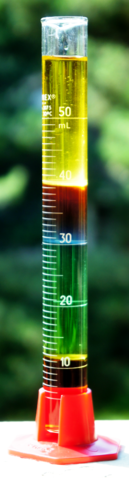Figure 1.16. Density column (from top to bottom) containing vegetable oil, wine, water, dish soap, and maple syrup. 

Density is an important physical parameter that explains why substances float on top of others (e.g., oil on top of water). When different substances are mixed that have appreciably different densities, those with lower densities will rise to the top, as shown in Figure 1.16.

Water is one of the few substances whose solid state has a smaller density than its liquid form. This explains why ice floats on top of liquid water.

How is the density of an irregularly shaped object determined? There’s no equation to calculate its volume, as there is for a cube, cylinder, sphere, etc. The method by water displacement is a technique often used. The object is placed into a container of water, whose initial volume is known. The object consequently displaces an amount of water equivalent to its volume.

In the simulation below, move the horizontal scroll bars to observe the relationships between density, mass and volume. You can also drag the blocks until they're fully submerged to 'measure' the volume of water displaced.

Q1.42

The volume of water displaced equals which of the following?

A

The sum of the initial and final volumes

B

The difference between the initial and final volumes

That’s right, the volume of the object = Vfinal – Vinitial = ∆V.

Density then becomes:

Q1.43

A 3.24 g object is immersed into a graduated cylinder filled with water. Use the following figure to determine the object’s density. Enter just the numerical value without units.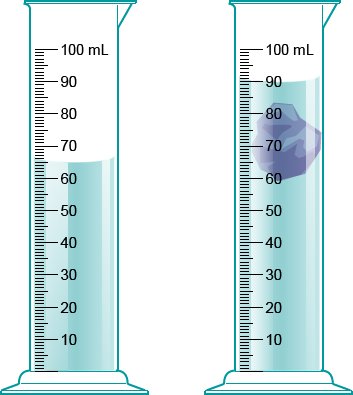### Tips for Dimensional Analysis

Example:
San Francisco and Los Angeles are 385 miles apart. Assuming an average speed of 65 miles per hour, how long will it take for you to drive between the cities?

Given: 385 miles
Target: hours (“how long will it take”)
“Per” always means a ratio.

• Any equivalence statement forms two conversion factors, which are reciprocals of each other.
• Equivalence statements may be given directly as an equality or less directly in the form of a sentence, for example, “65 miles per hour” could be given instead of as a ratio.
• Only multiplication and division are used in dimensional analysis! If units do not cancel, you most likely have to use the reciprocal of one of your conversion factors.
• Exact numbers derived from counting (not requiring a measuring tool) or from definitions are ignored for significant figure purposes.
• For example, 1 in/2.54 cm is ignored for the purposes of determining significant figures because it is not a measured quantity, but 65 miles/hr is not because it is a measured quantity (likely from a speedometer or radar gun). The latter restricts a multiplication or division step to two significant figures.

## 1.9 Counting Atoms: The Concept of the Mole

Now that we have learned about common measurement units and scientific notation, we can tackle a unit of measurement unique to chemistry: the mole. The microscopic, or particulate-level drawings introduced in earlier in this chapter clearly indicate individual atoms as spheres. However, it is not practical for us to quantify matter atom by atom in the laboratory. Instead, we are accustomed to quantifying matter at the macroscopic level, i.e., in bulk quantities. For example, when you walk into the balance room in a general chemistry lab, you will see that your balance measures quantities in grams, not in atoms; the mole concept will allow you to use the mass you measure on a balance to determine the number of atoms you have! This section introduces us to the mole concept. In Chapter 2, we will learn how to convert between the mass of a sample measured on a balance, and the number of atoms or molecules in that sample. For now we will focus on converting between moles and the number of atoms or molecules in a sample.

Named after Italian scientist Amedeo Avogadro, Avogadro’s number is a conversion factor linking the microscopic to the macroscopic properties of matter.

1 mole (mol) = 6.022 × 1023 individual units

One mole of any substance contains exactly 6.022 × 1023 individual units of the substance.

1 mole of people = 6.022 × 1023 people

1 mole of grains of sand = 6.022 × 1023 grains of sand

In chemistry, individual units are commonly atoms and molecules:

1 mole of carbon atoms = 6.022 × 1023 carbon atoms

1 mole of water molecules = 6.022 × 1023 molecules of water

Using dimensional analysis and problem-solving skills from section, the following are ways to use Avogadro’s number as a conversion factor.

To convert from atoms or molecules to moles, divide by Avogadro’s number:

To convert from moles to atoms or molecules, multiply by Avogadro’s number:

### Tips for Converting Between Moles and the Number of Atoms

Memorize Avogadro’s number: 1 mol = 6.022 x 1023 atoms.

When asked to calculate atoms from moles, your answer will always be MUCH, MUCH larger! Don’t be surprised by extremely large answers for the number of atoms!

Q1.44

How many atoms of sulfur are contained in a 2.7 mole sample?

A

1.6 × 10$^{21}$ atoms

B

1.6 × 10$^{22}$ atoms

C

1.6 × 10$^{23}$ atoms

D

1.6 × 10$^{24}$ atoms

Q1.45

How many moles of chlorine are in a sample containing 4.5 × 10$^{14}$ chlorine atoms?

A

7.5 × 10$^{-8}$ mol

B

7.5×10$^{-9}$mol

C

7.5 × 10$^{-10}$ mol

D

7.5 × 10$^{-11}$mol

## 1.10 Energy and Chemistry

As stated at the beginning of the chapter, all fields within the natural and physical sciences study the interaction between matter and energy. Up until this point, we have focused on becoming acquainted with the properties and states of matter. We will now shift our attention to a discussion of energy that will lay the foundation for later chapters.

Energy is defined as the ability to do work or generate heat and light. You experience energy every day. For example, when you pick up a book you are working against the gravitational potential energy between the book and the Earth's center of gravity. As you move the book, you impart kinetic energy, the energy of motion. Of the two types of energy described above, only kinetic energy plays a major role in chemistry since atoms, ions, and molecules are so small they are minimally affected by gravity. The kinetic energy of molecules will play an important role in our understanding of chemical reactions (Chapter 12), as such we will define it here:

KE = 1/2 mv2 where m is the mass of the object in kilograms and v is the velocity in meters per second.

If you carefully consider the units of mass and velocity in the context of this equation, you will arrive at an important quantity: the Joule, which is equal to 1 kg•m2/s2; this is approximately the amount of energy to pick up an apple from a table.

In addition to kinetic energy, other important types of energy that you will encounter in chemistry are: electrostatic potential energy, thermal energy, and the energy associated with electromagnetic radiation, often referred to colloquially as "light energy." In Chapter 19, you will also encounter nuclear energy which is more properly the realm of physics, but which is covered in most chemistry courses since it relies on an understanding of atomic structure.

We will briefly introduce the most relevant types of energy in the sections below.

### 1.10.1 Electrostatic Potential Energy

If you have ever been "shocked" when touching a doorknob on a dry winter day, you have experienced electrical charge. Many objects are electrically charged depending on the relative number of protons and electrons they contain. In the example of static electricity above, it is likely that you became negatively charged from walking across a carpeted floor and picking up extra electrons from the atoms in the carpet. When you touch the doorknob, the electrons "jump" from your negatively charged hand to the positively charged doorknob. This illustrates an important fact about electrical charges: opposite charges are attracted to each other.

Another common experience with charge is "hat hair." When you remove a knit winter cap the wool or nylon material strips electrons from your hair leaving the individual strands positively charged. The reason your hair sticks up is because the positively charged strands repel each thus illustrating another important aspect of electrical charges: like charges repel each other.

You can calculate the electrostatic potential energy between two charged particles using the equation below:

​

In this equation, k = 8.99 x 109Nm2/C2. For our present purposes, we need only focus on the charges and distance.

• Since the charges are in the numerator of the equation, the larger the magnitude of the charges, the stronger the energy of attraction or repulsion will be.
• The magnitude of the electrostatic potential energy is inversely related to the distance between the charges. Charges that are far apart will experience weak attraction or repulsion, while charges that are close together will experience strong attraction or repulsion. You have probably felt the effects of an inverse distance relationship if you have every played with magnets: try to push two like poles together and the repulsion gets stronger as the magnets get closer, try to push two opposite magnetic poles together and they will "snap" together when they get close and the attraction gets stronger.

Q1.46

Click on the letter of the set(s) that you expect to exhibit attractive electrostatic interactions.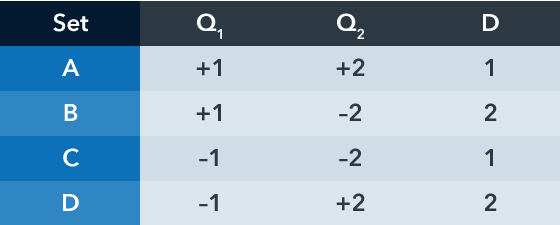Q1.47

Rank the sets of charge and distance data from strongest attraction (most negative) to weakest attraction (least negative).A

A,B,C,D

B

D,C,B,A

C

B,C,D,A

D

C,D,B,A

E

D,B,C,A

Electrostatic potential energy lies at the core of all chemical phenomena from atoms to bonding and reactivity. The reason atoms exist as they do is because the negatively charged electrons are attracted to the positive protons in the nucleus (Chapters 2 and 3). Ionic solids (i.e., salts) form because positive ions (cations) are attracted to negative ions (anions) and molecular compounds are held together by covalent bonds in which electrons are attracted to two or more atomic nuclei simultaneously (Chapters 4 and 5). It is important that you understand and remember the basic principles of electrostatic potential energy as you move forward in your study of chemistry.

### 1.10.2 Thermal Energy and Chemical Bonds

You are likely familiar with the heat generated by a gas burning stove through the process of combustion. The heat from combustion is a manifestation of breaking and making chemical bonds. For example, when methane (CH4) reacts with molecular oxygen (O2) the C–H bonds and O=O bonds break in order to form H–O bonds and C=O bonds.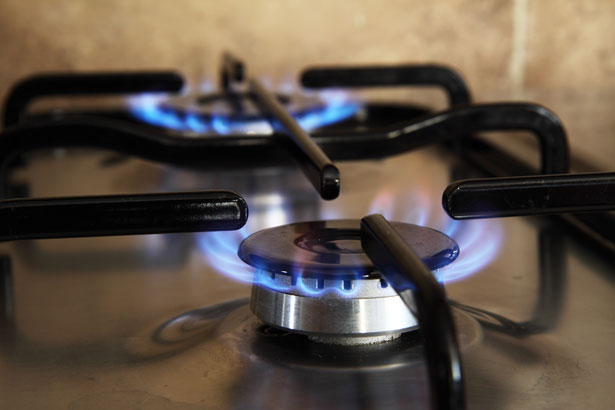Figure 1.17. Gas-burning stove and chemical reaction of methane combustion. 

Because the net result is the formation of stronger bonds, we say that thermal energy (aka heat) is released during this process. For every 16 grams of methane that reacts with oxygen, 891,000 Joules of thermal energy are released.

The thermal energy stored in chemical bonds plays a critical role in our daily lives as it drives everything from the respiratory and metabolic processes necessary for life to the internal combustion engines that facilitate modern transportation. You will learn more about the thermal energy stored in chemical bonds in Chapters 5, 12, and 13 .

### 1.10.3 Energy and Light

You have probably used a microwave oven to heat up left overs. When you open the door to remove your food, you do not typically experience intense heat, but when you go to take the first bite of food you may burn your mouth. How can the oven be cool while your food is painfully hot? You used the energy of electromagnetic radiation (i.e., light) to heat your food! The electromagnetic radiation from the microwave generator housed in your microwave oven caused the water molecules in your food to rotate rapidly thereby generating heat in your food but not in the air around it.

Electromagnetic radiation, which is immaterial (i.e., does not have mass, nor does it take up space), interacts with matter in myriad ways. For example, infrared radiation causes chemical bonds to vibrate which gives chemists useful information about the types of bonds in a molecule. Additionally, ultraviolet (UV) radiation has enough energy to break chemical bonds. This is the reason it is so important to use sunscreen; it protects the bonds in our DNA from uncontrolled scission, which can lead to mutations and ultimately skin cancer.

In chemistry the interaction between electromagnetic radiation in matter is used to great effect in the field of spectroscopy which allows scientists to probe the structure of atoms, ions, and molecules which are too small to be seen using conventional microscopy.

We will take a detailed look at the interaction between electromagnetic radiation and atoms in Chapter 3 as we learn about the early experiments that allowed chemists and physicists to elucidate the structure of the atom.

Q1.48

Match the example on the left with the correct type of energy on the right.

Premise
Response
1

X-ray imaging

A

Thermal Energy

2

Wrinkly clothes from clothes dryer

B

Electrostatic Potential Energy

3

Calories burned during exercise

C

Electromagnetic Energy

### Case Study: Significant Figures

So, just how important is it to pay attention to units and significant figures in your calculations? On a chemistry exam, it may very well mean the difference between grade tiers. However, as we are about to see, ignoring such attributes of problem-solving in the real world can mean the difference between life and death, between success and failure, and between astronomically large sums of money.Figure 1.20. The Gimli Glider after landing in Gimli, Manitoba, Canada, viewed from the front. 

In 1983, an Air Canada flight was forced to make an emergency landing after having run out of fuel. In 1970, Canada had switched to the metric system, and this aircraft was the first of Air Canada’s fleet to use metric measurements. Before takeoff, the aircraft’s fuel gauge was not working. The round crew was forced to manually calculate the amount of fuel required for the plane’s flight. Erroneously, pounds instead of kilograms was used in their calculations. The result: the plane had only half the required amount of fuel. Fortunately for all on board, the pilots were able to glide the aircraft to safety to a nearby airfield in Gimli, Manitoba, Canada. Hence, the aircraft was nicknamed the “Gimli Glider.”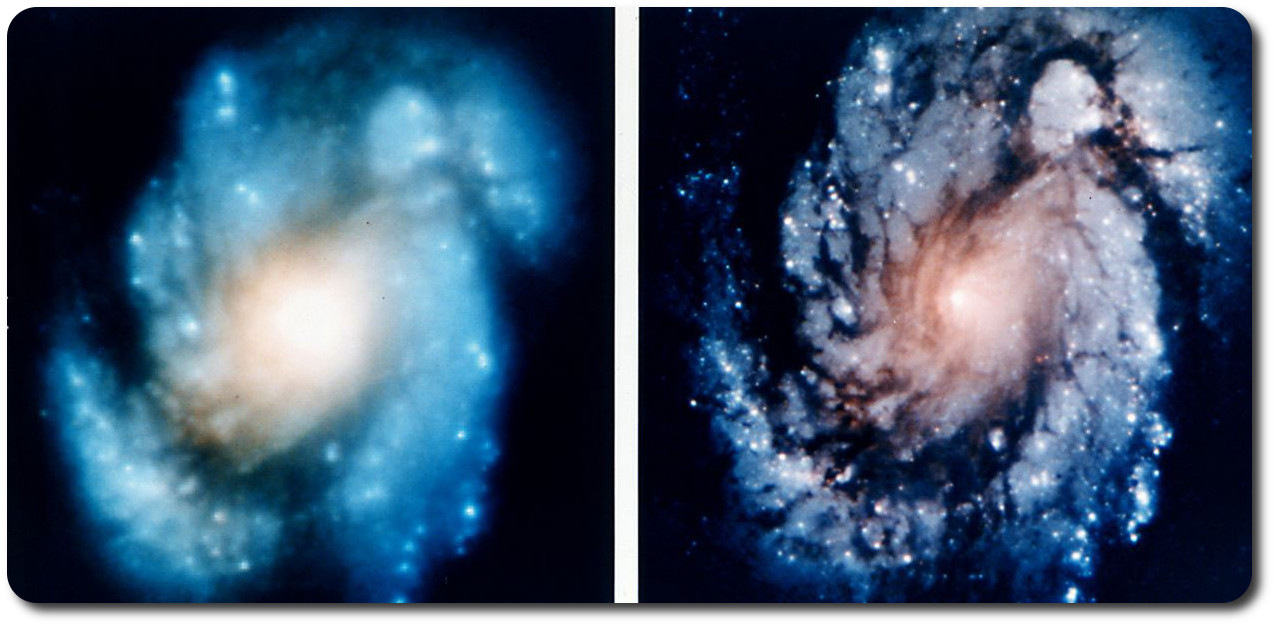Figure 1.21. Images of the M100 galaxy 55 million light years from Earch before (left) and after (right). Costar modification in 1993. 

The Hubble Space telescope is world-renown for its detailed, beautiful images of far away galaxies and cosmic events. Launched by NASA in 1990, Hubble’s first series of images had turned out blurry. The culprit: the telescope’s main mirror was too flat by 2.2 μm (1/50th the diameter of a human hair strand). Although this does not seem significant, the mirror had to be fixed 3 years later. Called the Corrective Optics Space Telescope Axial Replacement (Costar), the hardware modification cost around $8 million USD.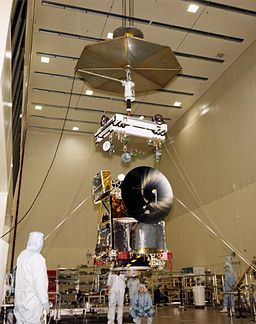Figure 1.22. Assembly of the Mars Climate Orbiter in 1998. ​​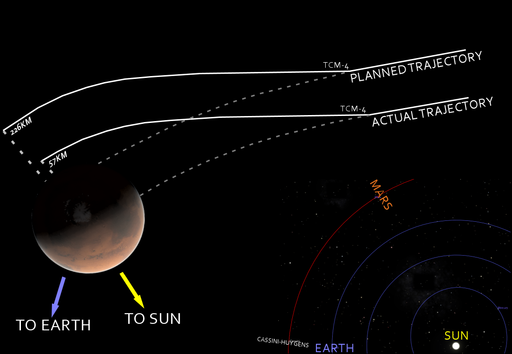Figure 1.23. Planned and actual trajectories of the Mars Climate Orbiter.  The Mars Climate Orbiter was planned to be the first-ever interplanetary weather satellite. Sent to Mars by NASA in December 1998, the total cost of the orbiter was$125 million USD.

However, in September 1999, NASA had lost communication with the orbiter. The culprit: both the SI and English system for altitude units were mistakenly used. An aerospace contractor in the United States had used the English system, while NASA had used the SI system for altitude. The flawed calculations had sent the orbiter dangerously too close to the Martian surface. Intended to orbit the red planet at 226 km high, the orbiter had approached at 57 km high, where 80 km was thought to have been the minimum safe orbital altitude. It is believed that the orbiter disintegrated due to atmospheric forces.

## Summary

• Matter typically exists in one of three states: solid, liquid, or gas.
• Matter can undergo physical and chemical changes.
• The scientific method is a cyclical process that is used to identify, investigate, and solve problems.
• Scientific notation is used to express very small or large quantities.
• Prefixes are attached to units to express very small or large scales.
• Percent error is often used to express how close a measurement is to a known value.
• Significant figures relate to the precision of a measurement.
• Dimensional analysis utilizes conversion factors to express one unit in terms of another.
• Density is the ratio of a substance’s mass to its volume.
• The mole is a specific unit used by chemists that allows mass to be related to the number of atoms, ions, or molecules in a macroscopic sample.
• Kinetic energy, electrostatic potential energy, thermal energy, and light energy are the most relevant forms of energy in chemistry.
• Like charges repel, opposite charges attract, and the strength of repulsion and attraction depends on the distance between the charges.
• Thermal energy is stored in chemical bonds. When bonds are made, energy is released in the form of heat.
• Light (electromagnetic radiation) energy interacts with matter and these interactions allow us to study the structure and properties of matter that is too small to see with optical microscopes.

## End of Chapter 1​This Content is Locked
Only a limited preview of this text is available. You'll need to sign up to Top Hat, and be a verified professor to have full access to view and teach with the content.

## Image Credits

 Image courtesy of Top Hat.

 Image courtesy of W. Oelen under CC BY-SA 3.0.

 Image courtesy of Victor Blacus under CC BY-SA 4.0.

 Image courtesy of Acdx under CC BY-SA 3.0.

 Image courtesy of Chris 73 under CC BY-SA 3.0.

 Image courtesy of Andy Beecroft under CC BY-SA 2.0.

 Image courtesy of Bruce McAdam under CC BY-SA 2.0.

 Image adapted from diagram by Whatiguana under CC-BY-SA-4.0.​

 Image courtesy of Kelvinsong under CC BY-SA 3.0.

 Image courtesy of PublicDomainPictures under CC0 1.0.

 Image courtesy of Mk2010 under CC BY-SA 3.0.

 Image courtesy of Dcoetzee under CC BY-SA 3.0.

 Images courtesy of NASA in the Public Domain.

 Image courtesy of NASA in the Public Domain.

 Image courtesy of Xession under CC BY 3.0.